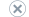# Lesson 5: Defining Relationships Between Dimensions and Measure Groups

Applies to:SQL Server Analysis ServicesAzure Analysis ServicesPower BI Premium

In the previous lessons in this tutorial, you learned that database dimensions added to a cube can be used as the basis for one or more cube dimensions. In this lesson, you learn to define different types of relationships between cube dimensions and measure groups, and to specify the properties of these relationships.

Note

Completed projects for all of the lessons in this tutorial are available online. You can jump ahead to any lesson by using the completed project from the previous lesson as a starting point. See Analysis Services samples to download the sample projects that go with this tutorial.

This lesson contains the following tasks:

Defining a Referenced Relationship
In this task, you learn to link a dimension to a fact table indirectly through a dimension that is linked directly through a primary key-foreign key relationship.

Defining a Fact Relationship
In this task, you learn to define a dimension based on data in the fact table, and to define the dimension relationship as a fact relationship.

Defining a Many-to-Many Relationship
In this task, you learn to relate a fact to multiple dimension members through the definition of a many-to-many relationship between dimension tables and fact tables.

Defining Dimension Granularity within a Measure Group
In this task, you learn to modify the granularity of a dimension for a specific measure group.

## Next Lesson

Lesson 6: Defining Calculations